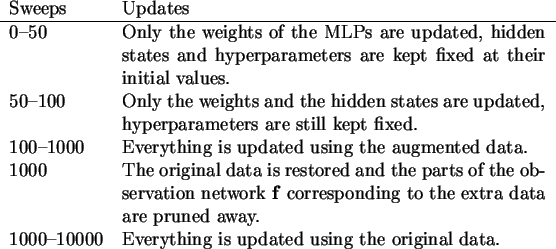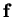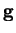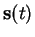Next: Learning with known state Up: Learning algorithm for the Previous: Evaluating and optimising the   Contents

## Learning procedure

The progress of learning in the switching NSSM is almost the same as in the plain NSSM. The parameters are updated in similar sweeps and the data are used in exactly the same way.

The HMM prototype means are initialised to have relatively small random means and small constant variances. The prototype variances are initialised to suitable constant values.

The phases in learning the switching model are presented in Table 6.2.In each sweep of the learning algorithm, the following computations are performed:

• The distributions of the outputs of the MLP networksandare evaluated as presented in Appendix B.
• The HMM state probabilities are updated as in Equation (6.14).
• The partial derivatives of the cost function with respect to the weights and inputs of the MLP networks are evaluated by inverting the computations of Appendix B and using Equations (6.37)-(6.39).
• The parameters for the continuous hidden statesare updated using Equations (6.36), (6.41) and (6.42).
• The parameters of the MLP network weights are updated using Equations (6.34) and (6.36).
• The HMM output parameters are updated using Equations (6.20) and (6.22), and the results from solving Equations (6.23)-(6.24).
• The hyperparameters of the HMM are updated using Equations (6.16) and (6.17).
• All the other hyperparameters are updated using similar procedure as with the HMM output parameters.Next: Learning with known state Up: Learning algorithm for the Previous: Evaluating and optimising the   Contents
Antti Honkela 2001-05-30